Case Based Questions (MCQ)

Chapter 15 Class 10 Probability
Serial order wise

## A child’s game has 8 triangles of which 3 are blue  and rest are red, and 10 squares of which 6 are blue  and rest are red. One piece is lost at random.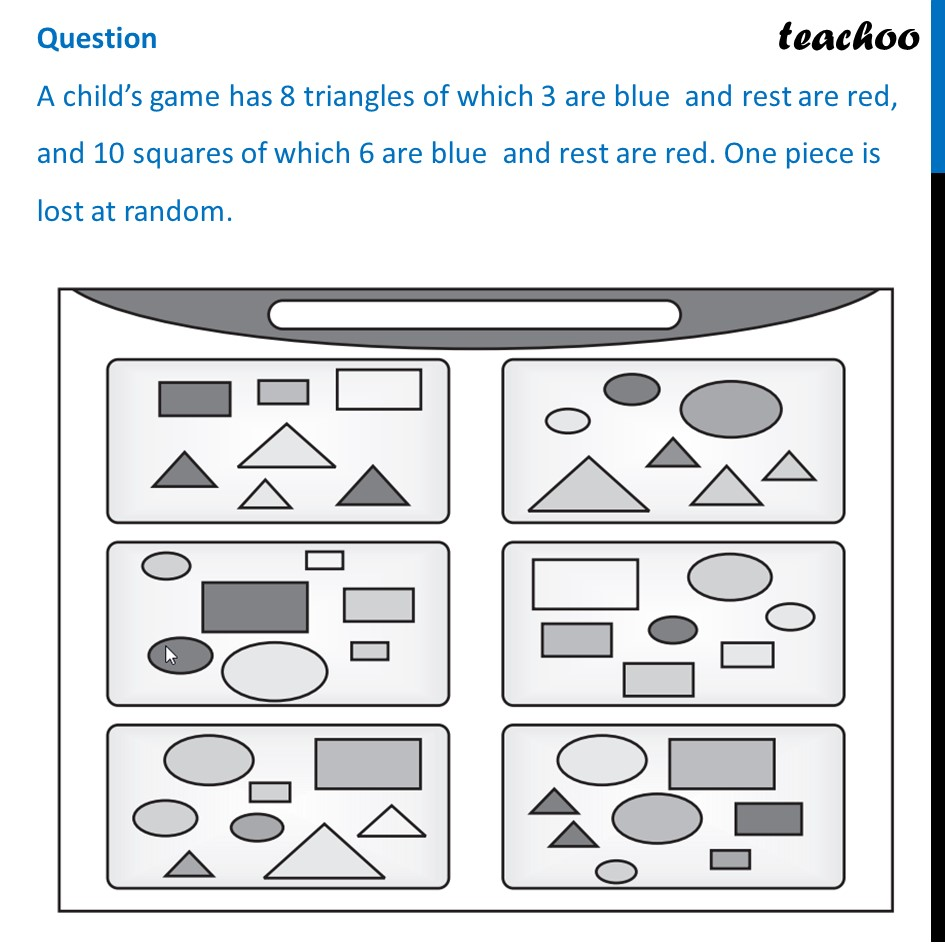## (c) 5, 5                                                (d) 8, 6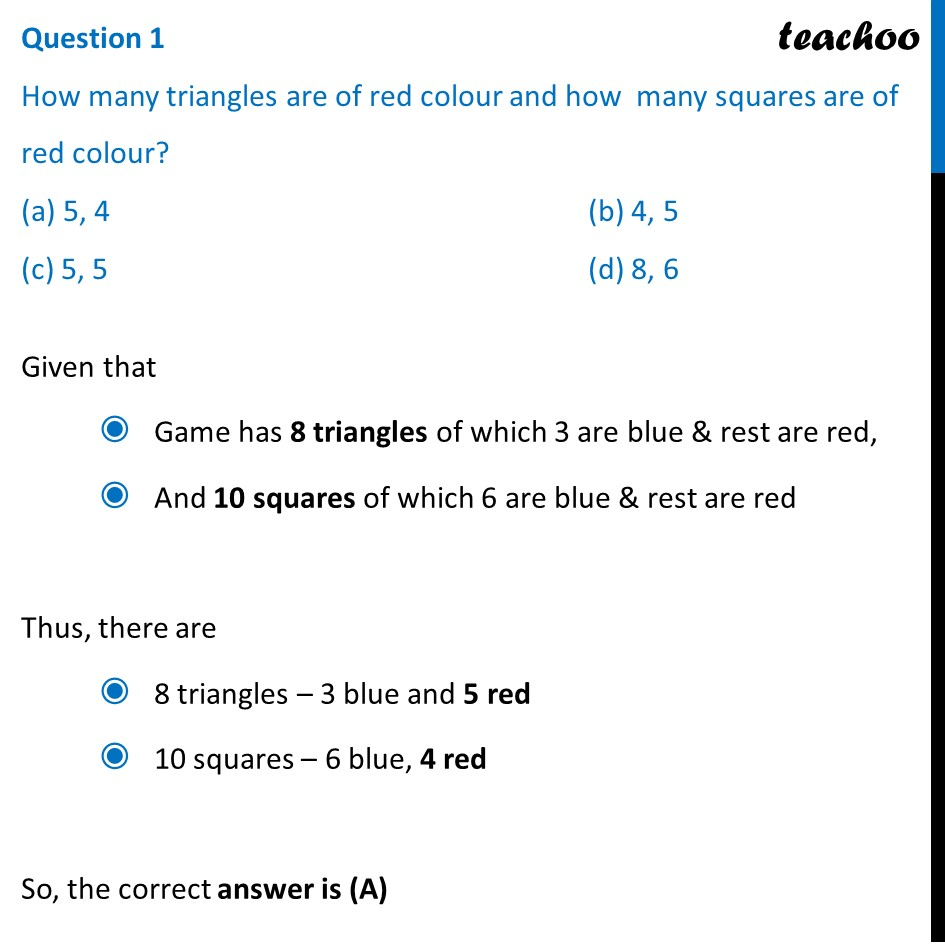## (C) 1/3                                          (D) 5/18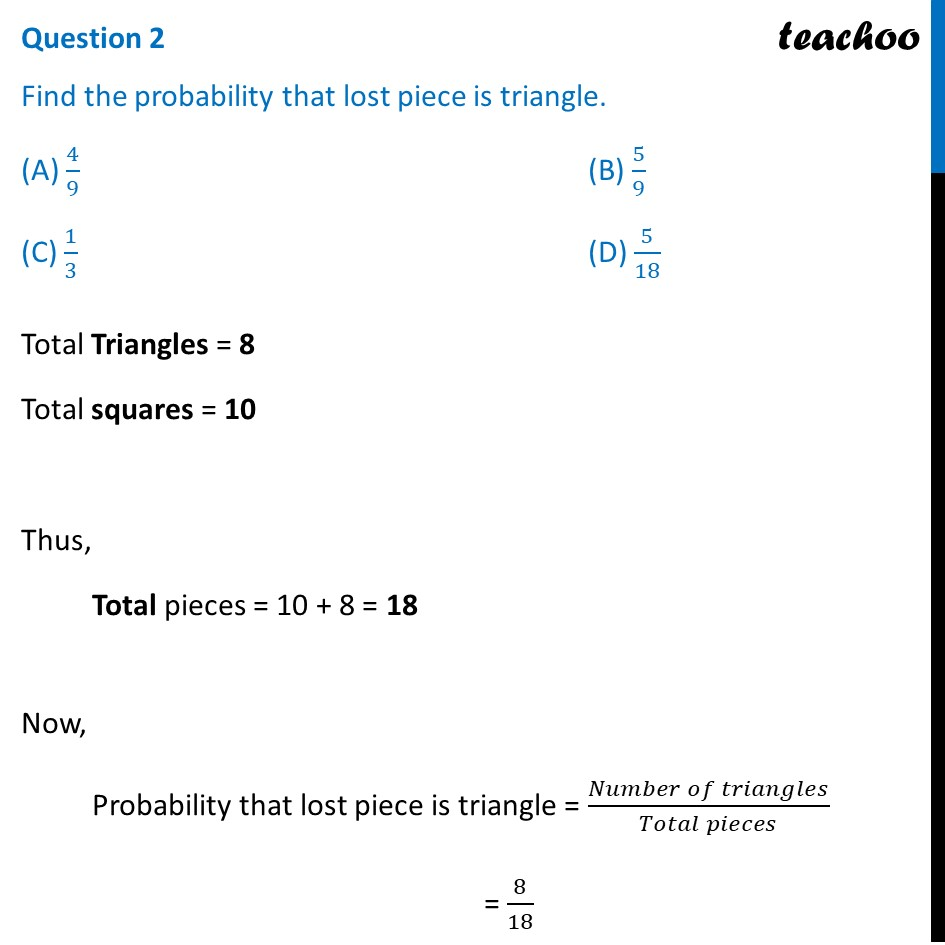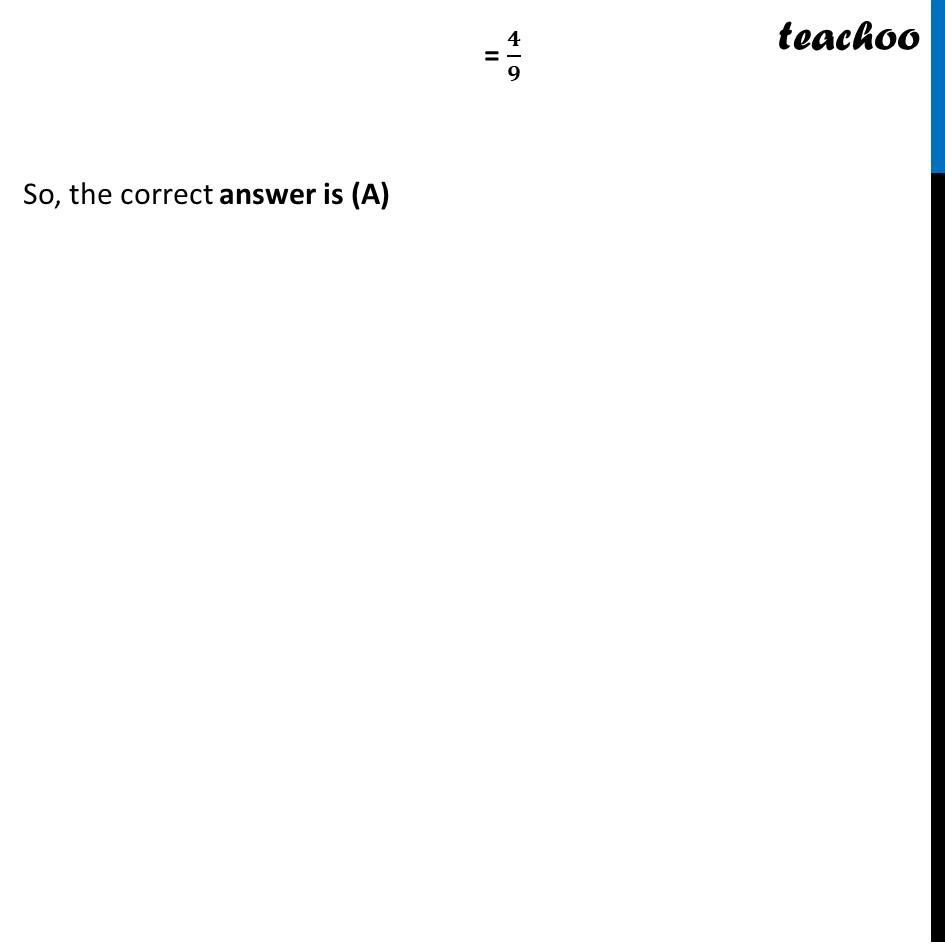## (C) 1/3                                        (D) 5/18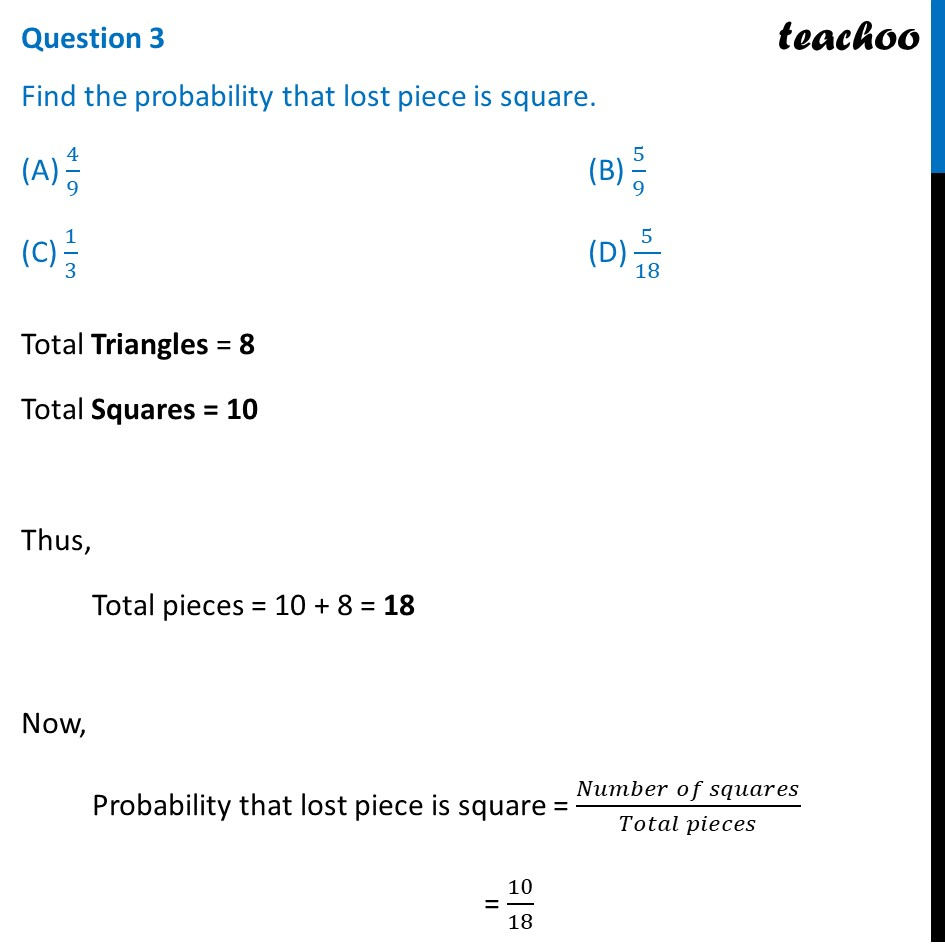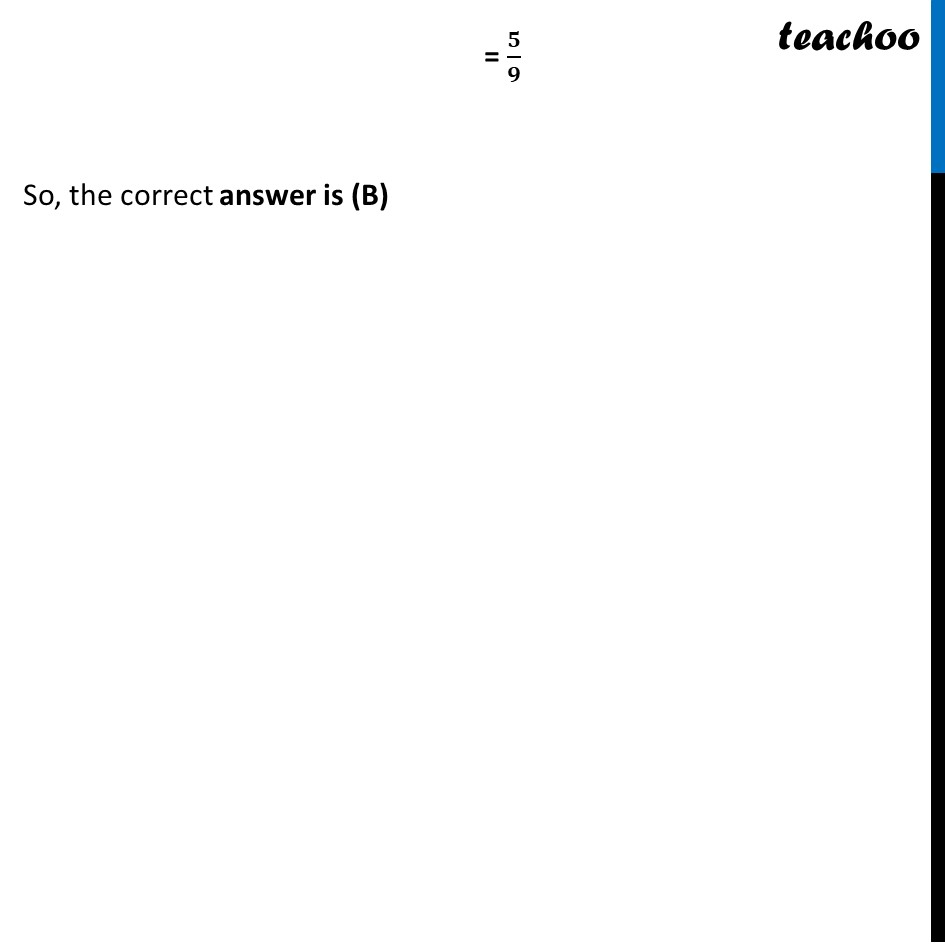## (C) 1/3                                                  (D) 5/18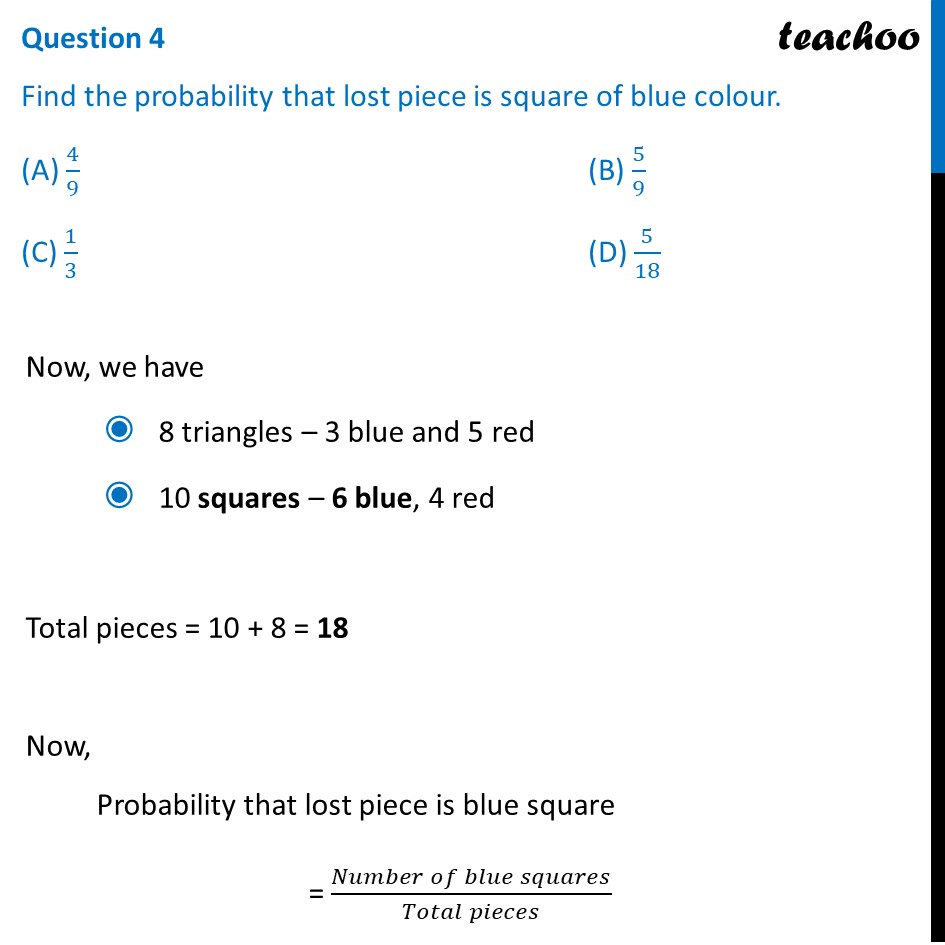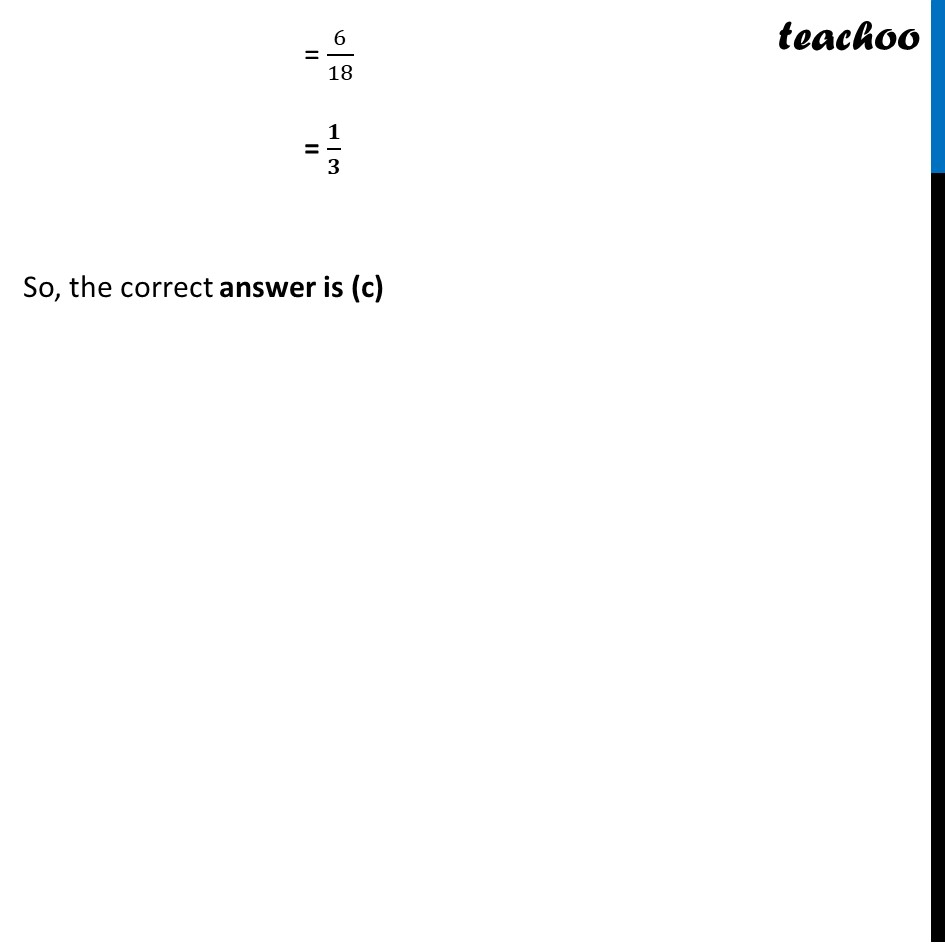## (C) 1/3                                                  (D) 5/18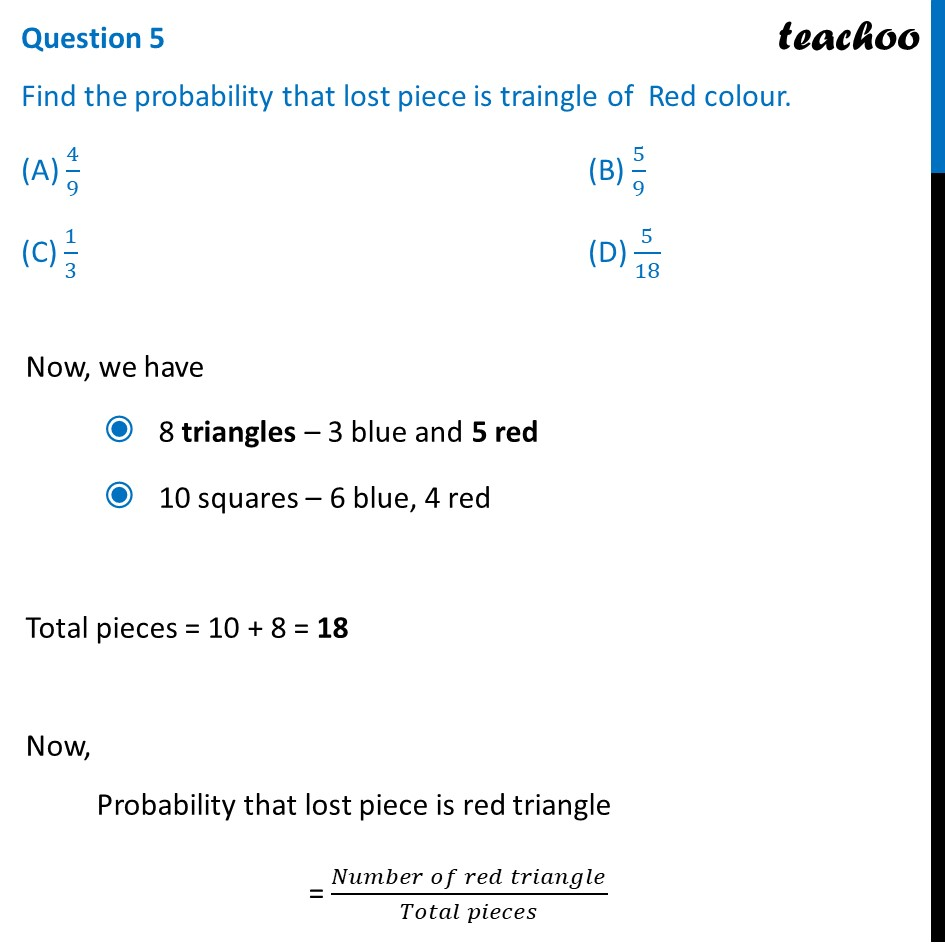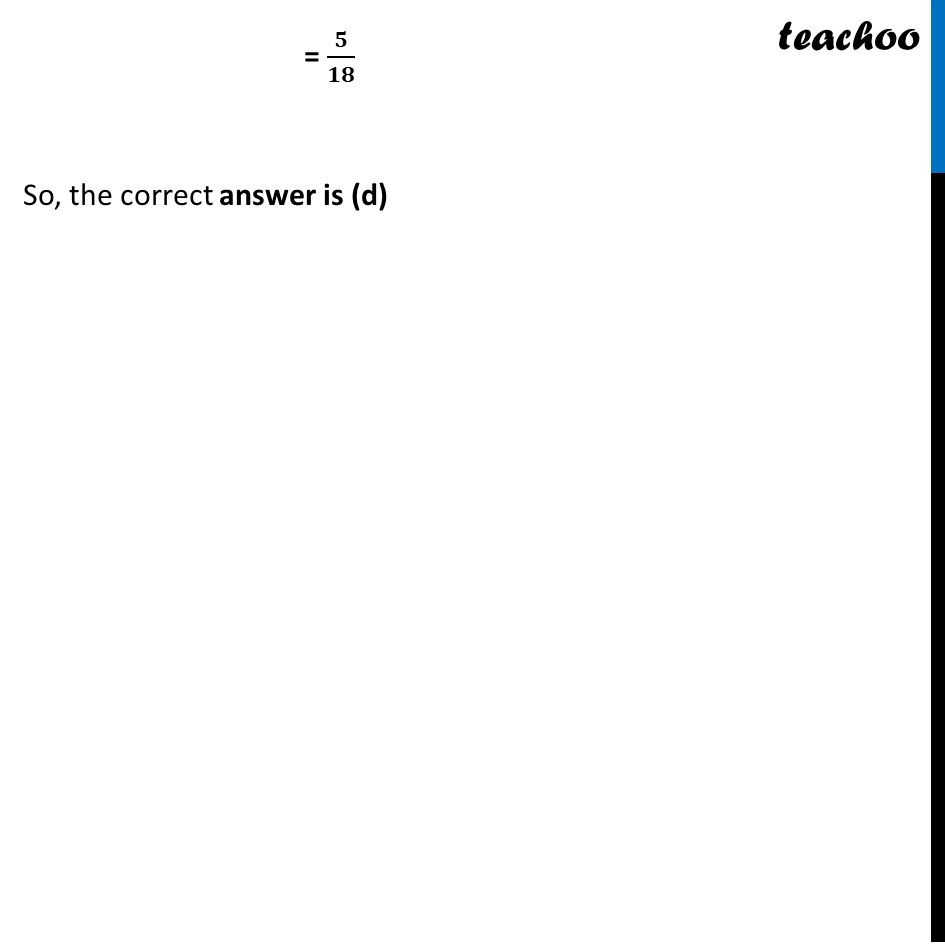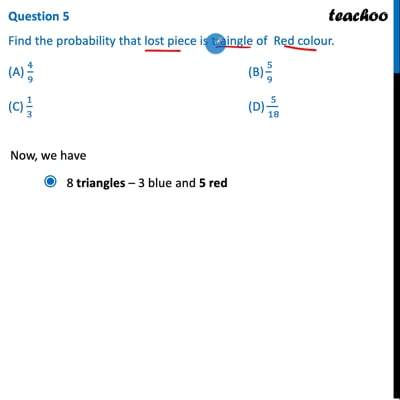This video is only available for Teachoo black users

Maths Crash Course - Live lectures + all videos + Real time Doubt solving!

### Transcript

Question A child’s game has 8 triangles of which 3 are blue and rest are red, and 10 squares of which 6 are blue and rest are red. One piece is lost at random. Question 1 How many triangles are of red colour and how many squares are of red colour? (a) 5, 4 (b) 4, 5 (c) 5, 5 (d) 8, 6 Given that Game has 8 triangles of which 3 are blue & rest are red, And 10 squares of which 6 are blue & rest are red Thus, there are 8 triangles – 3 blue and 5 red 10 squares – 6 blue, 4 red So, the correct answer is (A) Question 2 Find the probability that lost piece is triangle. (A) 4/9 (B) 5/9 (C) 1/3 (D) 5/18Total Triangles = 8 Total squares = 10 Thus, Total pieces = 10 + 8 = 18 Now, Probability that lost piece is triangle = (𝑁𝑢𝑚𝑏𝑒𝑟 𝑜𝑓 𝑡𝑟𝑖𝑎𝑛𝑔𝑙𝑒𝑠)/(𝑇𝑜𝑡𝑎𝑙 𝑝𝑖𝑒𝑐𝑒𝑠) = 8/18 = 𝟒/𝟗 So, the correct answer is (A) Question 3 Find the probability that lost piece is square. (A) 4/9 (B) 5/9 (C) 1/3 (D) 5/18Total Triangles = 8 Total Squares = 10 Thus, Total pieces = 10 + 8 = 18 Now, Probability that lost piece is square = (𝑁𝑢𝑚𝑏𝑒𝑟 𝑜𝑓 𝑠𝑞𝑢𝑎𝑟𝑒𝑠)/(𝑇𝑜𝑡𝑎𝑙 𝑝𝑖𝑒𝑐𝑒𝑠) = 10/18 = 𝟓/𝟗 So, the correct answer is (B) Question 4 Find the probability that lost piece is square of blue colour. (A) 4/9 (B) 5/9 (C) 1/3 (D) 5/18Now, we have 8 triangles – 3 blue and 5 red 10 squares – 6 blue, 4 red Total pieces = 10 + 8 = 18 Now, Probability that lost piece is blue square = (𝑁𝑢𝑚𝑏𝑒𝑟 𝑜𝑓 𝑏𝑙𝑢𝑒 𝑠𝑞𝑢𝑎𝑟𝑒𝑠)/(𝑇𝑜𝑡𝑎𝑙 𝑝𝑖𝑒𝑐𝑒𝑠) = 6/18 = 𝟏/𝟑 So, the correct answer is (c) Question 5 Find the probability that lost piece is traingle of Red colour. (A) 4/9 (B) 5/9 (C) 1/3 (D) 5/18Now, we have 8 triangles – 3 blue and 5 red 10 squares – 6 blue, 4 red Total pieces = 10 + 8 = 18 Now, Probability that lost piece is red triangle = (𝑁𝑢𝑚𝑏𝑒𝑟 𝑜𝑓 𝑟𝑒𝑑 𝑡𝑟𝑖𝑎𝑛𝑔𝑙𝑒)/(𝑇𝑜𝑡𝑎𝑙 𝑝𝑖𝑒𝑐𝑒𝑠) = 𝟓/𝟏𝟖 So, the correct answer is (d)